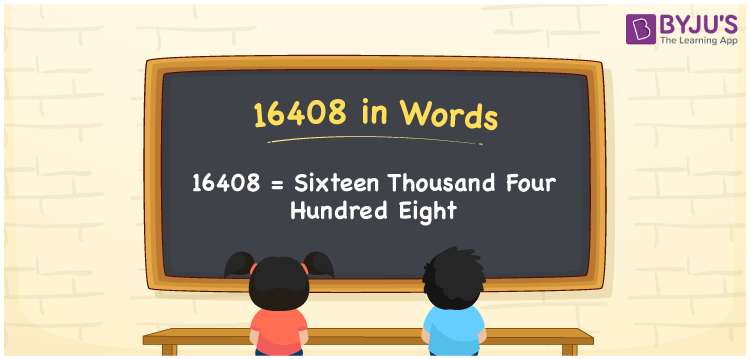# 16408 in words

16408 in words is written as Sixteen Thousand Four Hundred and Eight. In 16408, 1 has a place value of ten thousand, 6 is in the place value of thousand, 4 is in the place value of hundred, and 8 is in the place value of one. The article on Place Value gives more information. The number 16408 is used in expressions that relate to money, distance, length, social media views, and many more. For example, “There are Sixteen Thousand Four Hundred and Eight rupees as my savings.”

 16408 in words Sixteen Thousand Four Hundred and Eight Sixteen Thousand Four Hundred and Eight in Numbers 16408

## 16408 in English Words## How to Write 16408 in Words?

We can convert 16408 to words using a place value chart. This can be done as follows. The number 16408 has 5 digits, so let’s make a chart that shows the place value up to 5 digits.

 Ten thousand Thousands Hundreds Tens Ones 1 6 4 0 8

Thus, we can write the expanded form as:

1 × Ten thousand + 6 × Thousand + 4 × Hundred + 0 × Ten + 8 × One

= 1 × 10000 + 6 × 1000 + 4 × 100 + 0 × 10 + 8 × 1

= 16408.

= Sixteen Thousand Four Hundred and Eight.

16408 is the natural number that is succeeded by 16407 and preceded by 16409.

16408 in words – Sixteen Thousand Four Hundred and Eight.

Is 16408 an odd number? – No.

Is 16408 an even number? – Yes.

Is 16408 a perfect square number? – No.

Is 16408 a perfect cube number? – No.

Is 16408 a prime number? – No.

Is 16408 a composite number? – Yes.

## Solved Example

1. Write the number 16408 in expanded form

Solution: 1 x 10000 + 6 x 1000 + 4 x 100 + 0 x 10 + 8 x 1

We can write 16408 = 10000 + 6000 + 400 + 00 + 8

= 1 x 10000 + 6 x 1000 + 4 x 100 + 0 x 10 + 8 x 1.

## Frequently Asked Questions on 16408 in words

Q1

### How to write the number 16408 in words?

16408 in words is written as Sixteen Thousand Four Hundred and Eight.
Q2

### Is 16408 divisible by 2?

Yes. 16408 is divisible by 2.
Q3

### Is 16408 a prime number?

No. 16408 is not a prime number.